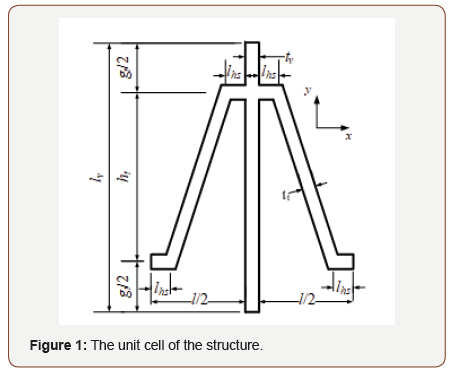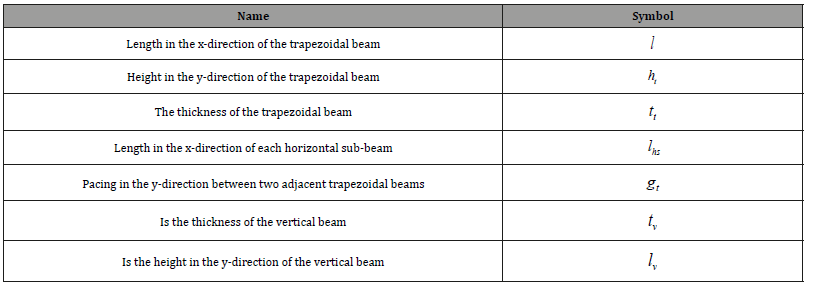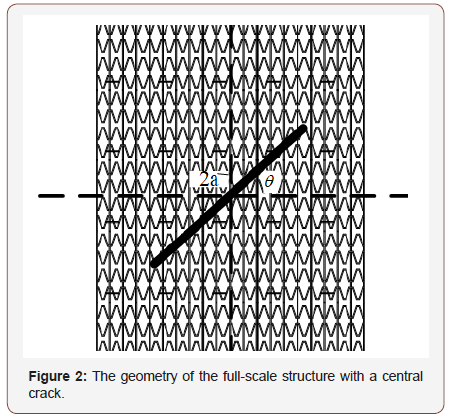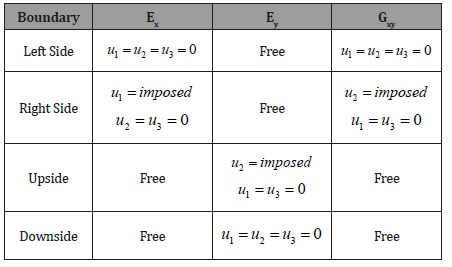# A Finite Element Study of The Elastic Properties of a Cracked Cellular Structure with Trapezoidal Beams

Jiong Zhang1, Weidong Liu2 and Liankun Wang1*

1School of Civil Engineering and Architecture, Wuyi University, PR China

2College of Energy and Electrical Engineering, Hohai University, PR China

Received Date: June 22, 2019;  Published Date: June 24, 2019

#### Abstract

The elastic properties of a cracked cellular structure of close-to-zero Poisson’s ratio with trapezoidal beams are studied by the finite element method in this paper. The effects of the crack length and crack angle on the non-dimensional equivalent modulus, including elastic moduli in the x-direction, y-direction, and the shear moduli are analyzed. The numerical results indicate that the degree of reduction in the non-dimensional equivalent modulus due to the crack depends on the crack angle and length.

Keywords:Cellular structure; Trapezoidal beam; Elastic moduli; Shear modulus; Finite element, Crack

#### Introduction

Wing morphing can drastically improve the mechanical and aerodynamic performance of the aircraft [1-3]. Flexible skin is very important to design morphing structures. The study of cell structures for flexible skin is of practical importance and has been a subject of interest for engineers and researchers.

In the past few decades, all kinds of cellular structures with positive and negative Poisson’s ratio have been proposed for flexible skins for its lightweight and high stiffness, such us conventional hexagonal honeycombs with positive Poisson’s ratios [4-9] and negative positive Poisson’s ratios [10-21]. However, nonzero Poisson’s ratio can unavoidably limit their applications on one-dimensional deformations. So, a lot of cellular structures with zero or close to zero positive Poisson’s ratios have been studied by researchers [22-26]. In the previous study, researchers employed the theoretical analysis, finite element methods, and experiments to study the elastic properties, including elastic moduli and shear moduli.

However, the flexible skins can inevitably contain cracks during service, which has bad effects on the mechanical properties of the flexible skins. However, there are few kinds of literature on the effects of the cracks on the mechanical properties of cellular structures. So, in this paper, the finite element method is used to study the elastic properties of a cracked cellular with close to zero positive Poisson’s ratios proposed by the authors . Some effective moduli are obtained in this study.

#### Geometrical Model

Figure 1 shows the unit cell of the proposed structure. The unit cell possesses parallel aligned vertical beams and trapezoidal beams between the vertical beams. The detailed geometry of the unit cell can be determined by the following parameters in Table 1. The following non-dimensional geometrical parameters are also defined to study the elastic properties of the structure: h = ht / l (height-tolength ratio of the trapezoidal beam), t = tt/l (thickness-to-length ratio of the trapezoidal beam), g = gt/l (spacing-to-length ratio of the trapezoidal beams), μ = lhs /l (horizontal sub-beam proportion ratio along x-direction), η = v t/tt (vertical beam to trapezoidal beam thickness ratio ), b = bt/l (cell depth-to-length ratio).Table 1: Detailed geometry parameters of the unit cell.Full-scale models of 20x20 unit cells with a central crack is shown in Figure 2. As shown in Figure 2, the length of the crack is 2a, and the inclined angle of the crack is θ .#### Finite Element Modeling

A three-dimensional model is created in the commercial soft package ABAQUS. 8-node shell elements are used in this study. In this paper, the non-dimensional geometrical parameters were fixed as h =1, t = 0.1, g =1, η =1. The non-dimensional equivalent elastic modulus in the x-direction, y-direction and shear modulus is defined as Ex/ E, Ey/E , Gxy/E respectively. The effects of the crack length and crack angle on the non-dimensional equivalent modulus are studied. The moduli E and Gxy are then obtained as the ratios between the averaged stresses and the imposed strains. The homogenized stresses are calculated by averaging the reaction forces along the direction of the imposed displacement over the boundary. The boundary conditions are listed in Table 2.

Table 2: Boundary conditions on the FE models.#### Results and Discussion

The typical deform shapes of the cracked structure are shown in Figure 3.##### The effects of the crack length on the non-dimensional equivalent modulus

In this part, the inclined crack angle is fixed at 90°. The crack length ratio a /W is selected to be 0.125,0.25,0.375,0.5,0.625, where W is the half width of the structure. Figure 4 shows the effects of the crack length on the Ex/ E, Ey/E , Gxy/E and Gxy/E.

As we can see from Figure 4, crack length has a great effect on the non-dimensional equivalent modulus. All three equivalent modulus decrease with the increase of the crack length.##### The effects of the crack inclined angle on the nondimensional equivalent modulus

In this study, the crack length ratio a /W is fixed at 0.5. The inclined crack angle θ is selected to be 0° ,15° ,30° , 45° ,60° ,75° ,90° . Figure 5 shows the effects of the crack inclined angle on the Ex/ E, Ey/E, Gxy/E and Gxy/E.

It can be seen in Figure 5,Ex/ E Edecreases with the increase of the inclined angle of the crack while Ey/E increases with the increase of the inclined angle of the crack. However, it is complicated for Gxy/E. Gxy/E Efirstly, decreases with the increase of the inclined angle when θ it is less than 60°. When θ > 60° Gxy/E Eincreases with the increase of the inclined angle.#### Conclusion

In this paper, the finite element method is used to study the elastic properties of a cracked cellular structure of close-to-zero Poisson’s ratio with trapezoidal beams. The effects of the crack length and crack angle on the non-dimensional equivalent modulus, including elastic moduli, are analyzed. From the numerical example, we can see that the equivalent modulus decreases with the increase of the crack length, while the effect of the crack inclined angle on the equivalent modulus is complicated.

#### Acknowledgement

This work was supported by the National Natural Science Foundation of China [Grant number 51605140]; the Natural Science Foundation of Jiangsu Province [Grant number BK20150802]; the Fundamental Research Funds for the Central Universities [Grant number 2015B02914] and the innovation and strong school engineering fund of Guangdong Province (NO.2017KQNCX201) and Natural Science Foundation of Guangdong Province (No.2018A030313430).

#### Conflict of Interest

No conflict of interest.

Article Details
Citation
Keywords
Scroll to
Scroll to Top